# Chapter 4  Graphics with Matplotlib

Matplotlib is a large and sophisticated graphics package for Python written in object oriented style. However, a layer built on top of this basic structure called pyplot accesses the underlying package using function calls. We describe a simple but useful subset of pyplot here.

## 4.1  Line plots

### 4.1.1  Simple plots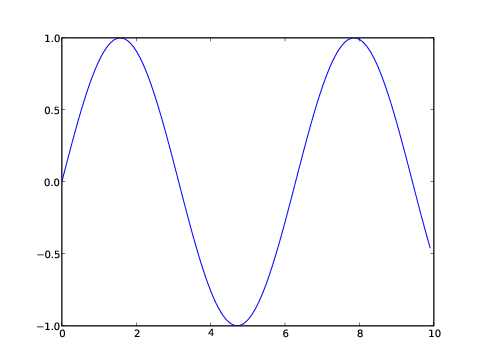Figure 4.1: A simple plot of a sine function.

Let’s type the following into Python:

```>>> from numpy import *
>>> import matplotlib.pyplot as plt
>>> x = arange(0.,10.,0.1)
>>> y = sin(x)
>>> ll = plt.plot(x,y)
>>> plt.show()
>>>
```

The first two lines import respectively the numpy and matplotlib pyplot modules. The second import is of a form we haven’t seen before. If we had typed `import matplotlib.pyplot`, all pyplot functions would need to be prefixed by the cumbersome `matplotlib.pyplot`. If on the other hand, we had used `from matplotlib.pyplot import *`, pyplot functions could have been used without any prefix. However, this would have left open the possibility that pyplot functions might clash with functions from other modules. The form used allows pyplot functions to be prefixed with the relatively short word `plt`. The next two lines define the arrays `x` and `y` which we want to plot against each other. The command `plt.plot(x,y)` performs this action, but we don’t see the result displayed on the screen until we type `plt.show()`. The result is shown in figure 4.1.

Calls to most pyplot functions return stuff that can sometimes be used in subsequent functions. That is why we assign these return values to variables, e. g., as in `ll = plt.plot(x,y)` in the above script, even though we don’t use these values here. An advantage of doing this is that the output is not cluttered up with obscure return values.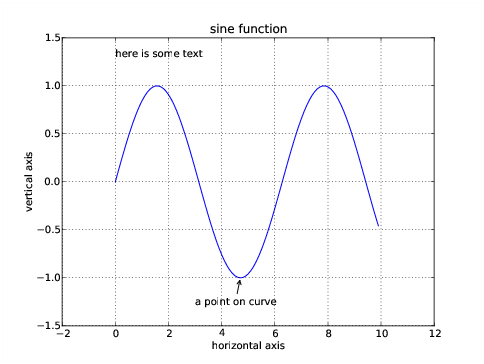Figure 4.2: Adding annotations to the plot.

Text such as a title, labels, and annotations can be added to the plot between the `plt.plot` and the `plt.show` commands. We can also change the axes if we don’t like the default choice and add grid lines to the plot:

```>>> ll = plt.plot(x,y)
>>> xl = plt.xlabel('horizontal axis')
>>> yl = plt.ylabel('vertical axis')
>>> ttl = plt.title('sine function')
>>> ax = plt.axis([-2, 12, -1.5, 1.5])
>>> grd = plt.grid(True)
>>> txt = plt.text(0,1.3,'here is some text')
>>> ann = plt.annotate('a point on curve',xy=(4.7,-1),xytext=(3,-1.3),
... arrowprops=dict(arrowstyle='->'))
>>> plt.show()
>>>
```

The resulting plot is shown in figure 4.2. The argument to the `axis` command is a list containing the lower and upper values of the x axis followed by the same for the y axis. The `text` command allows one to put arbitrary text anywhere in the plot. The `annotate` command connects text with plot points by an arrow. There are many arrow properties; we show only the simplest case here. The operation of the x and y label commands is clear.

### 4.1.2  Multiple lines and points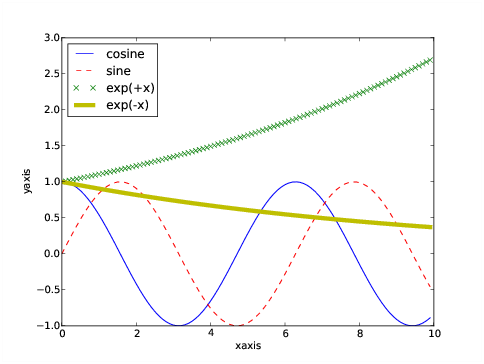Figure 4.3: Plot showing different line types and colors as well as a legend.

More complicated plots with multiple lines and line types can be made:

```>>> x = arange(0.,10,0.1)
>>> a = cos(x)
>>> b = sin(x)
>>> c = exp(x/10)
>>> d = exp(-x/10)
>>> la = plt.plot(x,a,'b-',label='cosine')
>>> lb = plt.plot(x,b,'r--',label='sine')
>>> lc = plt.plot(x,c,'gx',label='exp(+x)')
>>> ld = plt.plot(x,d,'y-', linewidth = 5,label='exp(-x)')
>>> ll = plt.legend(loc='upper left')
>>> lx = plt.xlabel('xaxis')
>>> ly = plt.ylabel('yaxis')
>>> plt.show()
>>>
```

The third argument of the plot command is an optional string, the first character of which is a color; one of "`b`", "`g`", "`r`", "`c`", "`m`", "`y`", "`k`", and "`w`" – mostly obvious, except "`k`" indicates black. The second and following characters indicate the line type. Types "`-`", "`--`", "`-.`", and "`:`" indicate solid, dashed, dash-dotted, and dotted lines respectively. Other types indicate markers: "`.`", "`x`", "`+`", "`o`", and "`*`" are fairly obvious. There are numerous other choices as well. In addition to the positional arguments, there is a large list of keyword arguments to the plot command. Perhaps the most useful is the linewidth, which is given in points.

The label argument in the plot command is used later by the legend command, which draws a legend in the specified location. Location choices are strings of the form `'upper left'`, `'lower center'`, `'right'`, etc. Alternatively, the loc argument may be omitted and Matplotlib puts the legend where it sees fit.

The resulting plot is shown in figure 4.3.

## 4.2  Log plots and histograms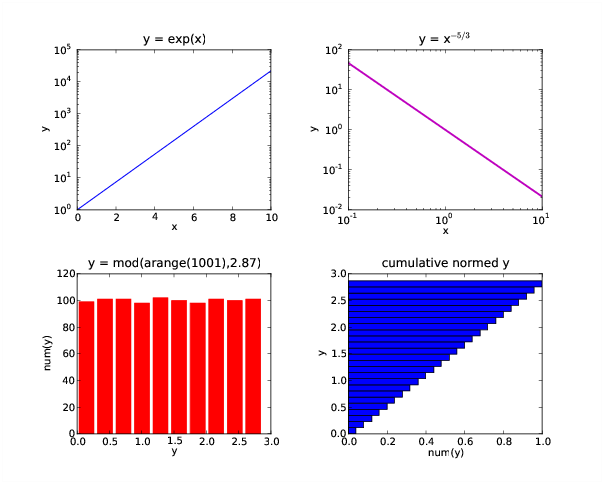Figure 4.4: Semi-log and log-log plots as well as two types of histogram plots.

A variety of other one-dimensional plotting modes are available in Matplotlib. The following script illustrates logarithmic plots and histograms:

```#!/usr/bin/python
#
# variety.py -- Make a variety of plots in a single figure

from numpy import *
import matplotlib.pyplot as plt

fg = plt.figure(figsize=(10,8))

sp = plt.subplot(2,2,1)
x = linspace(0,10,101)
y = exp(x)
l1 = plt.semilogy(x,y,color='m',linewidth=2)
lx = plt.xlabel("x")
ly = plt.ylabel("y")
tl = plt.title("y = exp(x)")

sp = plt.subplot(2,2,2)
y = x**-1.67
l1 = plt.loglog(x,y)
lx = plt.xlabel("x")
ly = plt.ylabel("y")
tl = plt.title("y = x\$^{-5/3}\$")

sp = plt.subplot(2,2,3)
x = arange(1001)
y = mod(x,2.87)
l1 = plt.hist(y,color='r',rwidth = 0.8)
lx = plt.xlabel("y")
ly = plt.ylabel("num(y)")
tl = plt.title("y = mod(arange(1001),2.87)")

sp = plt.subplot(2,2,4)
l1 = plt.hist(y,bins=25,normed=True,cumulative=True,orientation='horizontal')
lx = plt.xlabel("num(y)")
ly = plt.ylabel("y")
tl = plt.title("cumulative normed y")

plt.show()
```

The semi-log and log-log plots are largely self-explanatory. The plot command semilogx places the log scale along the x axis. The histogram plots are similarly simple. The rwidth option specifies the width of the histogram bars relative to the bin size, with a default of 1. The cumulative option plots a cumulative distribution and normed causes the integral of the distribution to be unity in the non-cumulative case. In the cumulative case the last histogram bar has a length of unity.

## 4.3  Two-dimensional plots

We now learn how to make two-dimensional plots of various kinds. These plots are created from two-dimensional NumPy arrays. In these arrays the second dimension (the column index) corresponds to the horizontal axis of the plot while the first dimension (the row index) corresponds to the vertical axis. Thus, arrays are plotted the same way they are printed, except that plots go from the bottom up while printing goes from the top down.

### 4.3.1  Contour plotsFigure 4.5: A simple contour plot with labels.

Here is the simplest way to make a contour plot:

```>>> x = linspace(0,10,51)
>>> y = linspace(0,8,41)
>>> (X,Y) = meshgrid(x,y)
>>> a = exp(-((X-2.5)**2 + (Y-4)**2)/4) - exp(-((X-7.5)**2 + (Y-4)**2)/4)
>>> c = plt.contour(x,y,a)
>>> l = plt.clabel(c)
>>> lx = plt.xlabel("x")
>>> ly = plt.ylabel("y")
>>> plt.show()
>>>
```

The result is shown in figure 4.5. The first four lines in the above script create the two-dimensional array `a`, which is then contoured `contour(x,y,a)`. The `x` and `y` are optional in this command, but if they are omitted, the axes default the the range [0,1].

The contour command doesn’t produce contour labels on its own; this action is provided by `clabel(c)`. Note that the value returned by the contour function is needed by the clabel function to generate the proper labels.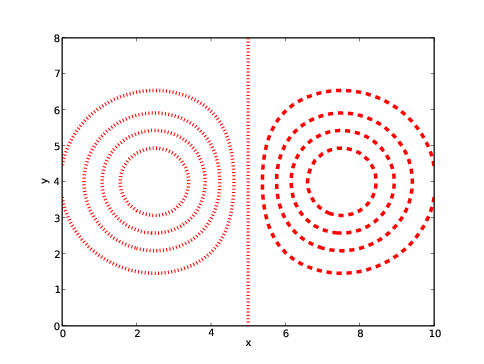Figure 4.6: An unlabelled contour plot in red with thick dotted lines and contour interval 0.2.

A sequence (tuple or list) of values can be used to define the levels contoured. Furthermore, various keyword arguments can be used to modify the presentation. The keyword colors can be used to define the color of the contours (using the color values indicated previously) and the keyword linewidths can be used to specify the width of lines. The style of lines can be set by the keyword linestyles, taking the possible values solid, dashed, dashdot, and dotted:

```>>> c = plt.contour(x,y,a,linspace(-1,1,11),colors='r',linewidths=4,
... linestyles='dotted')
>>> lx = plt.xlabel("x")
>>> ly = plt.ylabel("y")
>>> plt.show()
>>>
```

The result is shown in figure 4.6. Notice that regardless of the specified line style, Matplotlib insists on making negative contours dashed as long as a single color is specified! A solution is to fool Matplotlib into thinking multiple colors are being requested, by, for instance, specifying `colors=('r','r')` the the call to contour. This mechanism can also be used to (truly) specify different contour colors as well as different line widths and styles for different contours. The specified values are applied in sequence from negative to positive contours, repeating as necessary.

### 4.3.2  Filled contours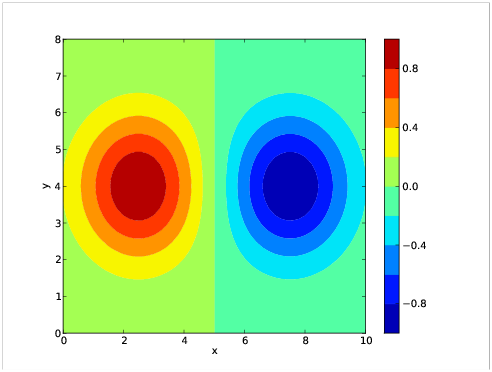Figure 4.7: A contour plot with filled contours and a color bar.

An alternate type of contour plot fills the gaps between contours:

```>>> c = plt.contourf(x,y,a,linspace(-1,1,11))
>>> b = plt.colorbar(c, orientation='vertical')
>>> lx = plt.xlabel("x")
>>> ly = plt.ylabel("y")
>>> ax = plt.axis([0,10,0,8])
>>> plt.show()
>>>
```

The result is shown in figure 4.7. The `colorbar()` command shown produces the labeled color bar on the right side of the plot. If `orientation='horizontal'`, the color bar appears below the plot.

### 4.3.3  Color mesh plots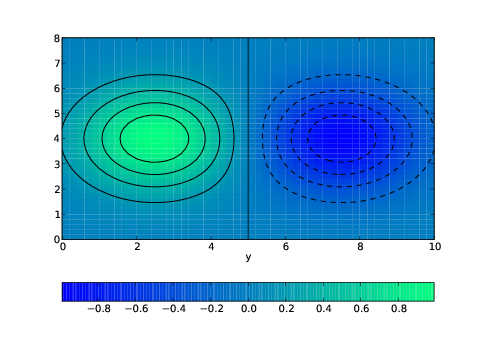Figure 4.8: A pcolor plot with a color bar and black contours. The winter color map is used.

The function pcolor produces a plot similar to that produced by contourf except that the color distribution is continuous rather than discrete. For example:

```>>> ac = 0.25*(a[:-1,:-1] + a[:-1,1:] + a[1:,:-1] + a[1:,1:])
>>> c = plt.pcolor(x,y,ac)
>>> d = plt.colorbar(c,orientation='horizontal')
>>> q = plt.winter()
>>> e = plt.contour(x,y,a,linspace(-1,1,11),colors='k')
>>> lx = plt.xlabel("x")
>>> ly = plt.xlabel("y")
>>> plt.show()
>>>
```

The first statement of this script computes grid-centered values of the array `a`. This is strictly necessary to align the plot properly with the axes, since pcolor centers its pixels on grid boxes whereas contour and contourf assume that `a` is defined on grid edges. However, for very small small grid boxes the half-grid displacement may be insignificant.

### 4.3.4  Vector plots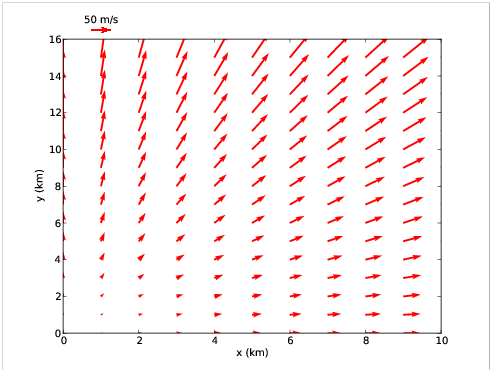Figure 4.9: A vector plot with a legend.

Vector plots can also be made in Matplotlib. Here is a script producing a vector plot and a key showing the scale of the vectors:

```>>> x = linspace(0,10,11)
>>> y = linspace(0,15,16)
>>> (X,Y) = meshgrid(x,y)
>>> u = 5*X
>>> v = 5*Y
>>> q = plt.quiver(X,Y,u,v,angles='xy',scale=1000,color='r')
>>> p = plt.quiverkey(q,1,16.5,50,"50 m/s",coordinates='data',color='r')
>>> xl = plt.xlabel("x (km)")
>>> yl = plt.ylabel("y (km)")
>>> plt.show()
>>>
```

The quiver command produces vector plots from two-dimensional arrays (`u` and `v` in this case) containing the vector component values. The grid on which the vectors are plotted is defined by the first two arguments of quiver – the two-dimensional arrays `X` and `Y` in this case. However, quiver will accept the original one-dimensional axis vectore `x` and `y` as well. The color of the vectors is specified in the usual fashion with the color keyword.

The quiver arguments `angles='xy'` and `scale=1000` are very important. Setting the angles keyword to `'xy'` means that the vector components are scaled according to the physical axis units rather than geometrical units on the page. The actual scaling factor which multiplicatively converts vector component units to physical axis units is width/scale where width is the width of the plot in physical units and scale is the number specified by the scale keyword argument of quiver. In our example width = 10 km and scale = 1000, so vectors are plotted to a scale of 0.01 km / ( m / s). The angle keyword argument is not available in versions of Matplotlib earlier than 0.99, which is a serious omission.

The quiverkey command produces a legend consisting of a single vector and a label indicating how long it is in physical units. In order, the positional arguments are (1) the variable returned by the quiver command, (2) and (3) the x and y coordinates of the legend, (4) the length of the arrow in physical units, and (5) the legend label. The keyword argument coordinates tells Matplotlib which units define the legend location; the most useful are ’axes’, in which x and y range from 0 to 1 across the plot and ’data’ in which physical axis coordinates are used. As in the above example (which is shown in figure 4.9), the legend may be located outside of the actual plot.

### 4.3.5  Masked arrays and Matplotlib

Matplotlib honors the NumPy conventions for masked arrays, in that masked regions of two-dimensional plots are omitted.

The use of masked arrays with vector plots and filled contour plots is a bit buggy at this point. For vectors, it is best to eliminate masked arrays in favor of arrays which give vectors zero length in masked regions. Hopefully this situation will improve in subsequent version of Matplotlib.

## 4.4  Finer control of plotting, color, and text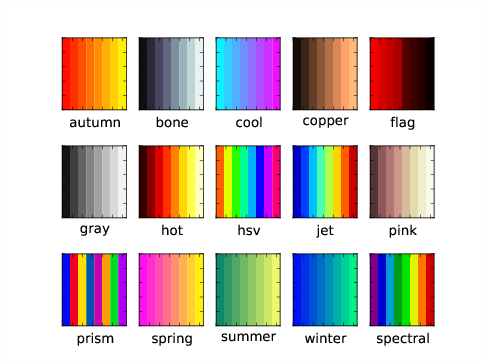Figure 4.10: The pre-defined colormaps of Matplotlib.

Figure 4.8 illustrates the use of an alternate color map, in this case, winter, generated by the `winter()` function. Colormaps provided by Matplotlib include autumn, bone, cool, copper, flag, gray, hot, hsv, jet, pink, prism, spring, summer, winter, and spectral and each has its associated function call. Figure 4.10 illustrates each of these colormaps. The default is jet.

An alternate way to set a color is by specifying its additive RGB value in a hex string. The hexadecimal digits are (1, 2, 3, ..., 9, a, b, c, d, f), so pure red is `'#ff0000'`, pure green is `'#00ff00'`, and pure blue is `'#0000ff'`, while black is `'#000000'` and white is `'#ffffff'`. With this scheme each primary color has 256 possible values, which is precisely the number that an eight bit unsigned integer can provide. So, for instance, bright yellow, which is a mixture of red and green, is specified by `color='#ffff00'`, and a darker red and a lighter blue would be obtained respectively from `color='#aa0000'` and `color='aaaaff'`.

Various global options can be set directly in the rcParams dictionary of Matplotlib, which contains default settings for many variables. Set any desired entries before invoking any other plotting commands. Since rcParams is a dictionary, the dictionary keys can be printed with the command `plt.rcParams.keys()` and the current value of a particular key (such as font.size) can be listed with `plt.rcParams['font.size']`.

The key font.size sets the default font size in plots. The default size of 12 pts is generally too small for presentation plots. Increasing this to 16 pts may be done with the command `plt.rcParams['font.size'] = 16`.

Increasing the font size can cause axis labels to overlap – Matplotlib is not (yet) smart enough to compensate for this. Axis numerical labeling can be changed using the xticks and yticks commands. (Recall that the xlabel and ylabel commands take care of the axis name labels.) For example, the command

```>>> tx = plt.xticks(linspace(5,25,5))
```

labels the x axis with the numerical values 5, 10, 15, 20, 25. In general, the argument of the xticks command is a list or array with the desired numerical labels, starting at the left end of the axis and ending at the right end. The yticks command works similarly for the y axis.

A similar problem can arise with colorbar labels, especially if the color bar is horizontally oriented. The solution here is a keyword argument to the colorbar command named ticks. So, for example, one could use

```>>> plt.colorbar(c,orientation='horizontal',ticks=linspace(-1,1,5))
```

to define the spacing of the labels on the color bar.

By default Matplotlib does some fancy footwork to eliminate line plotting commands that overlap other lines of the same type, and therefore would not be visible. However, this procedure can on occasion backfire, resulting in strange plotting behavior. If a problem of this type is suspected, this procedure can be turned off with the command

```>>> plt.rcParams['path.simplify'] = False
```

This command (like other assignments to rcParams) should be issued before any actual plotting commands.

## 4.5  Combination plots

### 4.5.1  Multiple plots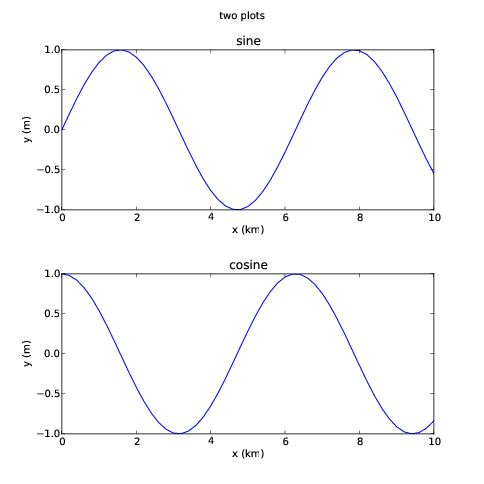Figure 4.11: Figure with two different plots.

Technically, before issuing any plotting commands, the figure command must be issued. However, pyplot takes care of this in simple cases with the default creation of a figure with dimensions 8 by 6 inches (when printed on paper). If different figure dimensions are desired, the figure command needs to be called explicitly with the figsize keyword argument.

Figures with multiple plots can be created using the subplot command. This command has three arguments, the number of rows of plots in the figure, the number of columns, and the particular plot being created next:

```>>> x = arange(0.,10.1,0.2)
>>> a = sin(x)
>>> b = cos(x)
>>> fig1 = plt.figure(figsize = (8,8))
>>> p1 = plt.subplot(2,1,1)
>>> l1 = plt.plot(x,a)
>>> lx = plt.xlabel("x (km)")
>>> ly = plt.ylabel("y (m)")
>>> ttl = plt.title("sine")
>>> p2 = plt.subplot(2,1,2)
>>> l2 = plt.plot(x,b)
>>> lx = plt.xlabel("x (km)")
>>> ly = plt.ylabel("y (m)")
>>> ttl = plt.title("cosine")
>>> sttl = plt.suptitle("two plots")
>>> plt.show()
>>>
```

The results are shown in figure 4.11. Notice that an “ubertitle” can be created using the suptitle command. Unfortunately, the font size of the ubertitle is less than the font size of the subplot titles. However, the font size of both title and ubertitle can be adjusted with the keyword parameter fontsize, e. g., `fontsize=16`.

The `plt.subplots_adjust(hspace=0.4)` command increases the height spacing of subplots from its smaller default value. This keeps the x axis label in the upper plot from overlapping with the title of the lower plot. Another optional keyword parameter for the subplots_adjust command is wspace, which solves similar problems for horizontal spacing. The use of the `plt.subplots_adjust` command is also illustrated in figure 4.4. Other options for `plt.subplots_adjust` are left, right, bottom, and top. These adjust overall plot margins. Multple options separated by commas can be used in a single `plt.subplots_adjust` call.

Matplotlib still has some rough edges when it comes to font size and plot spacing, but at least the tools to fix these problems are available!

### 4.5.2  Overlaying plots

Figure 4.7 demonstrates that line plots (e. g., plots produced by plot, contour, quiver, etc.) can be overlayed on a filled contour or a pcolor plot. In addition, line plots may be overlayed on each other. We can exert fine control over the order in which these are plotted using the zorder keyword option in these plotting commands. For instance including `zorder=0` in one plot and `zorder=5` in another makes the second plot appear on top of the first. Text always appears on top of all graphics.

## 4.6  Making plot output files

The call to show() in the above examples pops up a window with your plot. Various icons are displayed at the bottom of the window which allow you to manipulate the plot in various ways such as zooming in, changing the aspect ratio, etc. The right-most icon pops up a window which allows you to specify an output file for the plot. Various image formats are available as well as postscript, encapsulated postscript, and PDF.

## 4.7  Further information

The Matplotlib page at Sourceforge http://matplotlib.sourceforge.net/ is the primary reference for Matplotlib. This documents all plotting commands and has a link to a User Guide. The latest source code can also be downloaded here.

The version documented here is 0.99.1. As noted, a few serious omissions make versions earlier than 0.98 less useful for certain purposes.